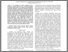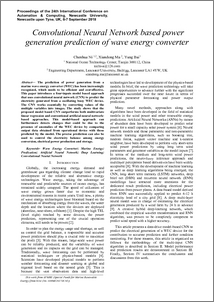# Convolutional Neural Network based power generation prediction of wave energy converter

Ni, Chenhua and Ma, Xiandong and Bai, Yang (2019) Convolutional Neural Network based power generation prediction of wave energy converter. In: Proceedings of the 24th International Conference on Automation and Computing. IEEE, pp. 460-465. ISBN 9781862203419Preview
PDF (p_177)
p_177.pdf - Accepted Version

## Abstract

The prediction of power generation from a marine wave energy converter (WEC) has been increasingly recognized, which needs to be efficient and cost-effective. This paper introduces a four-inputs model based approach that uses convolutional neural network (CNN) to predict the electricity generated from a oscillating buoy WEC device. The CNN works essentially by converting values of the multiple variables into images. The study shows that the proposed model based CNN outperforms both multivariate linear regression and conventional artificial neural network based approaches. This model-based approach can furthermore detects changes that could be due to the presence of anomalies of the WEC device by comparing output data obtained from operational device with those predicted by the model. The precise prediction can also be used to control the electricity balance among energy conversion, electrical power production and storage.

Item Type:
Contribution in Book/Report/Proceedings
Subjects:
Departments:
ID Code:
128118
Deposited By:
Deposited On:
11 Oct 2018 10:46
Refereed?:
Yes
Published?:
Published